This is an archival dump of old wiki content --- see scipy.org for current material.

The cookbook is a place for community contributions of recipes, howtos and examples.

Complete documentation and tutorials for matplotlib can be found at matplotlib's webpage

# Simple Plotting

• Sigmoidal Functions - plotting simple functions

•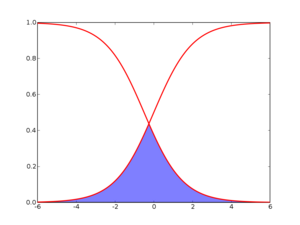• Multiline Plots - how to plot multiple lines over one another

•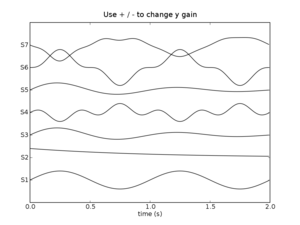• Bar Charts - how to make a bar chart

•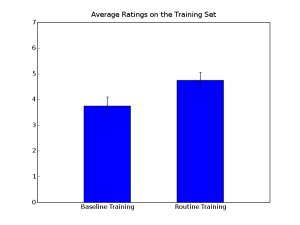• Common Errors - Compilation of common errors that can cause erroneous behavior. Check before emailing mailing lists.

• /Animations - how to animate your figures.

• Multicolored Line - different colors for different parts of a line

•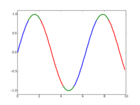• Shaded Regions - how to plot grey shaded regions using transparency.

•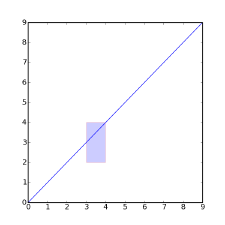• /Arrows - how to plot arrows

•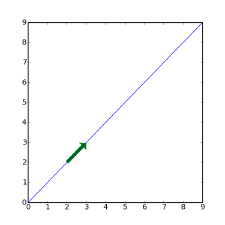• Unfilled Histograms - how to plot histograms that are un-filled and don't look like bar charts.

•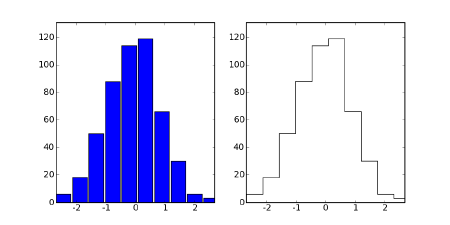• Cookbook/Histograms - 2D histograms with variable bin width.

•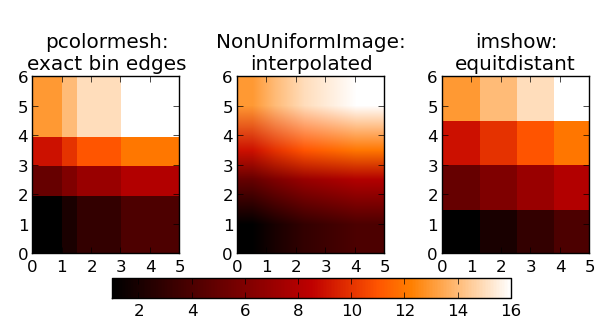• Custom Log Plot Labels - plotting log plots with custom tick labels that are formatted as integer numbers rather than exponents as is the default.

•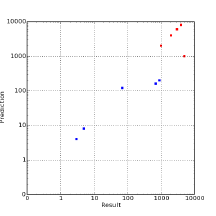• Thick Axes - how to make thick axes lines and bold fonts.

•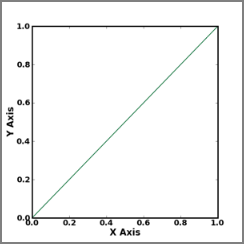• /Maps - how to plot data on map projections

•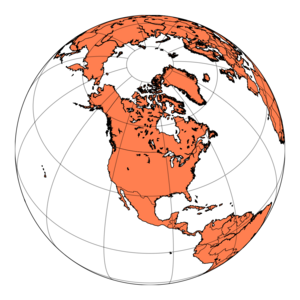• Plotting values with masked arrays - How to plot only selected values of an array, because some values are meaningless (detector malfunction), out of range, etc. etc.

• /Transformations - Using transformations to convert between different coordinate systems.

• TreeMap - classic treemap style plots

• Hinton Diagrams - A way of visualizing weight matrices

•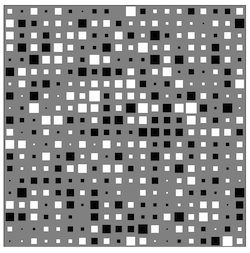# Typesetting

• Using TeX - formatting matplotlib text with LaTeX

•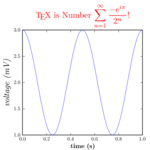• LaTeX Examples - Complete examples for generating publication quality figures using LaTeX.

# 3D Plotting

 NOTE: Experimental work has been going on to integrate 3D plotting functionality into matplotlib. Please see the related mplot3d documentation or take a look at matplotlib gallery for example 3D plots. For a more sophisticated 3D visualization and plotting interface, you can try Mayavi which is actively maintained and features an 'mlab' interface similar to matplotlib's 'pylab'.
• 3D plots - Simple 3D plots using matplotlibs built-in 3D functions (which were originally provided by John Porter's mplot3d add-on module).

•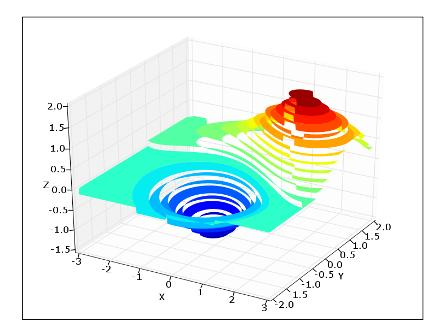• VTK Integration - How to import plots into VTK.

•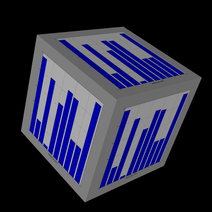# Misc

SciPy: Cookbook/Matplotlib (last edited 2015-10-24 17:48:26 by anonymous)